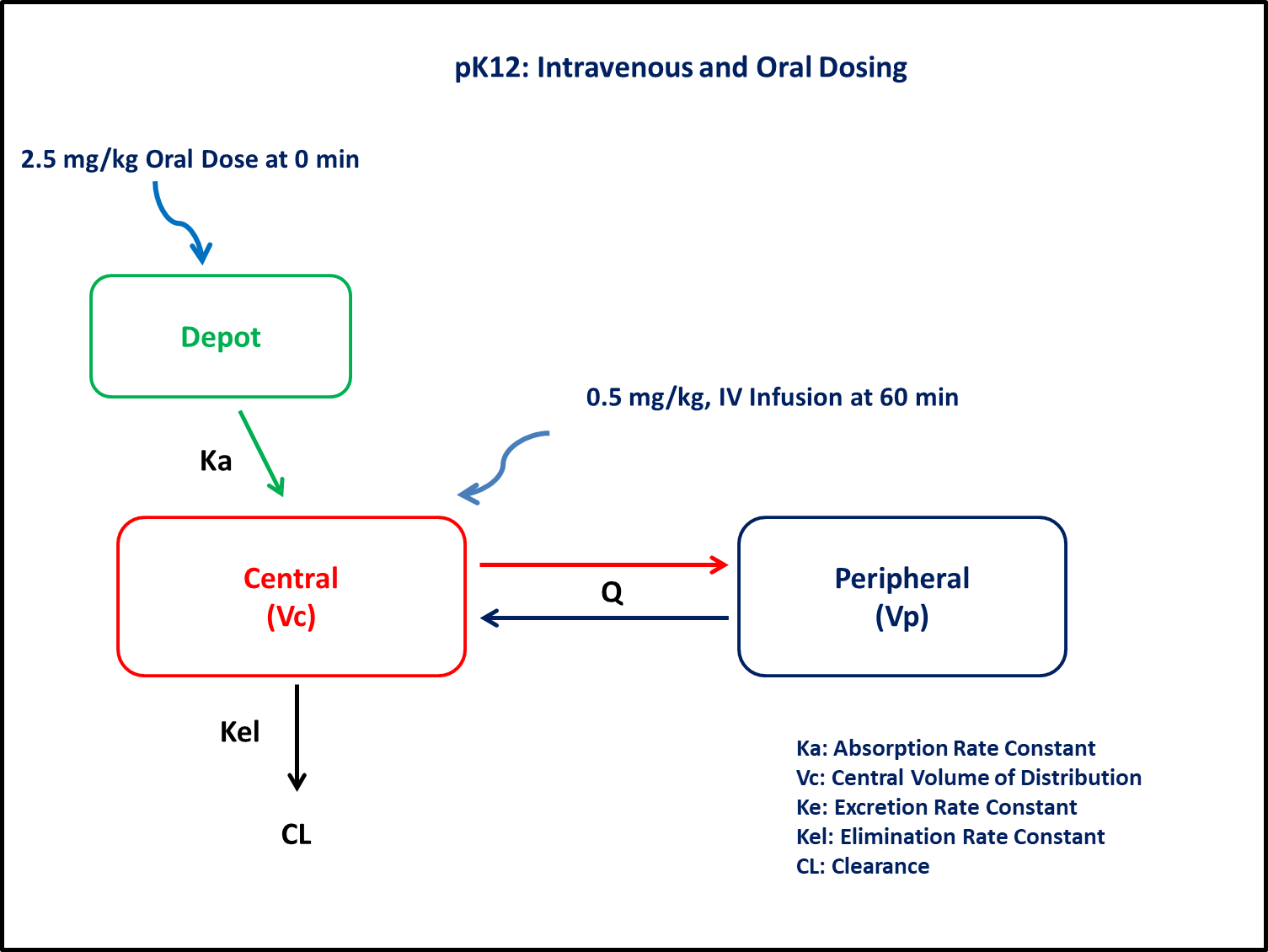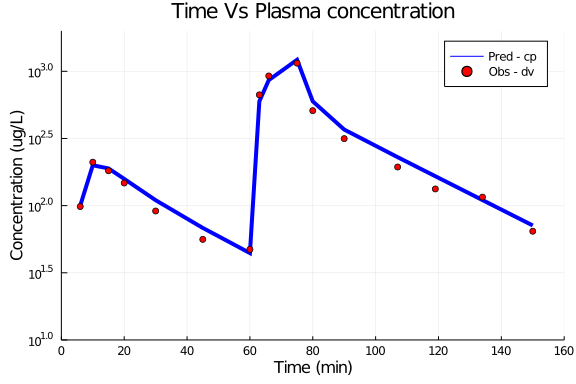# Exercise PK12 - Intravenous and oral dosing

### Background

To determine (oral) bioavailability, the drug is administered by both oral and intravenous route. The adminstration generaly is done in crossover manner at diffrent times separated by a washout period. But if the drug follows a time-dependant clearance (and if the washout period is long), then it may affect the results. To avoid this situation, the doses can be administered semi-simultaneously separated by a small time upto 1 hour.

In the current study, the test drug was administered oraly followed a constant rate infusion (reference) for 15 mins at 60 mins .

• Structural model - Two compartment model with first order absorption and elimination

• Route of administration - Oral and IV given simulataneously

• Dosage Regimen - 2.5 mg Oral and 0.5mg IV infusion of 15 mins

• Number of Subjects - 1### Learning Outcomes

This exercise will help to determine bioavailability of a compound administered semi-simultaneously by oral and intravenous route

### Objectives

• To bulid a two compartment model for semi-simultaneous oral and intravenous administeration

• To use final parameter estimates

• To design semi-simultaneous dosage regimen

• To simulate and plot a single subject with predefined time points.

### Libraries

call the "necessary" libraries to get started.

using Pumas
using Plots
using CSV
using StatsPlots
using Random


### Model

A two compartment model with oral absorption is build for a semi-simultaneous administration of oral dose followed by intravenous infusion.

pk_12           = @model begin
@param   begin
tvka        ∈ RealDomain(lower=0)
tvcl        ∈ RealDomain(lower=0)
tvq         ∈ RealDomain(lower=0)
tvvc        ∈ RealDomain(lower=0)
tvvp        ∈ RealDomain(lower=0)
tvlag       ∈ RealDomain(lower=0)
tvF         ∈ RealDomain(lower=0)
Ω           ∈ PDiagDomain(7)
σ²_prop     ∈ RealDomain(lower=0)
end

@random begin
η           ~ MvNormal(Ω)
end

@pre begin
Cl          = tvcl * exp(η)
Q           = tvq * exp(η)
Vc          = tvvc * exp(η)
Vp          = tvvp * exp(η)
Ka          = tvka * exp(η)
lags        = (Depot = tvlag * exp(η),)
bioav       = (Depot = tvF * exp(η),)
end

@dynamics begin
Depot'      = -Ka*Depot
Central'    =  Ka*Depot - (Cl/Vc)*Central - (Q/Vc)*Central + (Q/Vp)*Peripheral
Peripheral' =  (Q/Vc)*Central - (Q/Vp)*Peripheral
end

@derived begin
cp          = @. 1000*(Central/Vc)
dv          ~ @. Normal(cp, sqrt(cp^2*σ²_prop))
end
end

PumasModel
Parameters: tvka, tvcl, tvq, tvvc, tvvp, tvlag, tvF, Ω, σ²_prop
Random effects: η
Covariates:
Dynamical variables: Depot, Central, Peripheral
Derived: cp, dv
Observed: cp, dv


### Parameters

• Ka - Absorption Rate Constant (min⁻¹)

• Cl - Clearance (L/min/kg)

• Q - Inter-compartmental distribution (L/kg)

• Vc - Volume of Central Compartment (L/kg)

• Vp - Volume of Peripheral Compartment (L/kg)

• lag - Lag time (min)

• F - Bioavailability

• σ - Residual Error

param  = (tvka    = 0.103,
tvcl    = 0.015,
tvq     = 0.021,
tvvc    = 0.121,
tvvp    = 0.276,
tvlag   = 4.68,
tvF     = 0.046,
Ω       = Diagonal([0.0,0.0,0.0,0.0,0.0,0.0,0.0]),
σ²_prop = 0.04)

(tvka = 0.103, tvcl = 0.015, tvq = 0.021, tvvc = 0.121, tvvp = 0.276, tvlag
= 4.68, tvF = 0.046, Ω = [0.0 0.0 … 0.0 0.0; 0.0 0.0 … 0.0 0.0; … ; 0.0 0.
0 … 0.0 0.0; 0.0 0.0 … 0.0 0.0], σ²_prop = 0.04)


### Dosage Regimen

Dosage Regimen = 2.5 mg/kg oraly followed by 15 min intravenous infusion of 0.5 mg/kg starting from 60 mins after oral dosing administered to a single subject (sub1)

ev_oral = DosageRegimen(2.5, time=0, cmt=1)
ev_inf  = DosageRegimen(0.5, time=60, cmt=2, duration=15)
ev1     = DosageRegimen(ev_oral,ev_inf)
sub1    = Subject(id=1, events=ev1)

Subject
ID: 1
Events: 3


### Simulation

To simulate plasma concentration with the specific observation time points for a given dosage regimen 'DR'

Random.seed!(123)
sim_s1  = simobs(pk_12, sub1, param, obstimes = [6,10,15,20,30,45,60,63,66,75,80,90,107,119,134,150]);


### Dataframe & Plot

df1 = DataFrame(sim_s1)
dropmissing!(df1, :cp)
@df df1 plot(:time, :cp, yaxis=:log,
label= "Pred - cp", xlabel="Time (min)", ylabel="Concentration (ug/L)",
title= "Time Vs Plasma concentration", color=[:blue], linewidth=4,
xticks=[0,20,40,60,80,100,120,140,160], xlims=(0,160), ylims=(10,2000))
@df df1 scatter!(:time, :dv, yaxis=:log,
label="Obs - dv", color=[:red], markershape=[:circle])